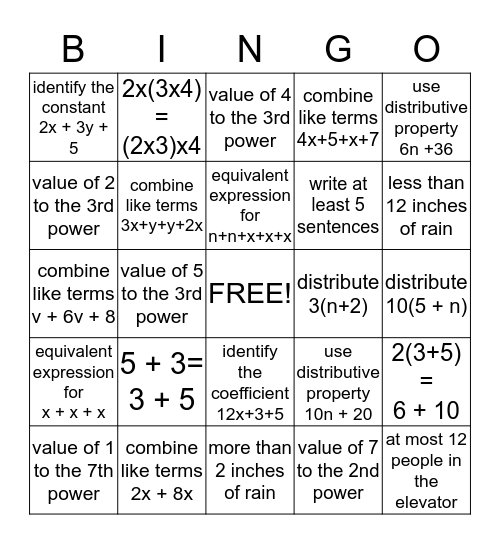# Algebra BingoThis bingo card has a free space and 24 words: 5 + 3= 3 + 5, combine like terms 2x + 8x, equivalent expression for n+n+x+x+x, value of 1 to the 7th power, use distributive property 6n +36, identify the constant 2x + 3y + 5, value of 2 to the 3rd power, 2x(3x4) = (2x3)x4, distribute 3(n+2), identify the coefficient 12x+3+5, write at least 5 sentences, combine like terms v + 6v + 8, use distributive property 10n + 20, 2(3+5) = 6 + 10, combine like terms 3x+y+y+2x, value of 7 to the 2nd power, distribute 10(5 + n), value of 4 to the 3rd power, less than 12 inches of rain, value of 5 to the 3rd power, at most 12 people in the elevator, equivalent expression for x + x + x, combine like terms 4x+5+x+7 and more than 2 inches of rain.

## Play Online